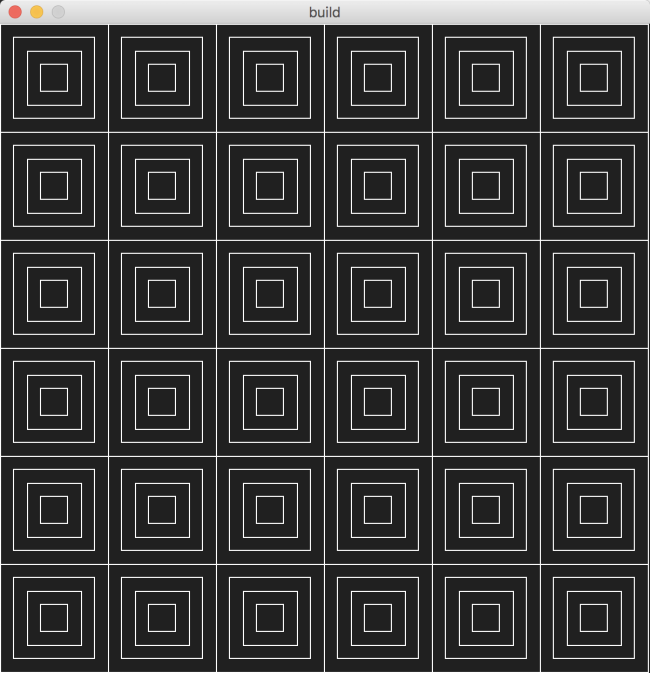#### Howdy, Stranger!

We are about to switch to a new forum software. Until then we have removed the registration on this forum.

# Animate Pattern

edited June 2017

Hi guys, I need your help! I am working on a simple exercise where I am trying to animate a pattern which I created from rectangles. What I want to achieve is to rotate each individual rectangle. I am feeling that I am very close but still no enough:-)) Probably I used translate() and pushMatrix() popMatrix(0 in a wrong place of my code. Thanks in advance for any help!Tagged:

• edited June 2017
``````    Here is my code --->

int num = 6;
sQuare[][] mysQuare = new sQuare[num][num];

void setup() {
size(602,602);

for (int i = 0; i < mysQuare.length; i ++) {
for (int j = 0; j < mysQuare.length; j ++) {

mysQuare[i][j] = new sQuare(100 * i, 100 * j, 100);
}
}
}

void draw() {
background(#202020);

for (int i = 0; i < mysQuare.length; i ++) {
for (int j = 0; j < mysQuare.length; j ++)  {
mysQuare[i][j].display();
mysQuare[i][j].rectRotate();
}
}
}

class sQuare {

float xpos;
float ypos;
int boxsize;
float theta = 0;
float speed = 0.005;

sQuare (float x, float y, int size) {

xpos = x;
ypos = y;
boxsize = size;
}

void display() {

pushMatrix();
translate(width/2, height/2);
for (int r = 1; r < 5; ++r) {

rotate(theta);
stroke(#FFFFFF);
strokeWeight(1);
noFill();
rectMode(CENTER);
rect(xpos + 50, ypos + 50, boxsize/4 * r, boxsize/4 * r);

}
popMatrix();
}

void rectRotate() {

theta += speed;
if (theta > 3.14 || theta < 0) {
speed = speed * -1;
}
}
}
``````
• Close enough. Have a look at this:

``````int num = 6;
sQuare[][] mysQuare = new sQuare[num][num];

void setup() {
size(602, 602);
for (int i = 0; i < mysQuare.length; i ++) {
for (int j = 0; j < mysQuare.length; j ++) {
mysQuare[i][j] = new sQuare(100 * i, 100 * j, 100);
}
}
}

void draw() {
background(#202020);
for (int i = 0; i < mysQuare.length; i ++) {
for (int j = 0; j < mysQuare.length; j ++) {
mysQuare[i][j].display();
}
}
}

class sQuare {

float xpos;
float ypos;
int boxsize;
float theta = 0;
float speed = 0.005;

sQuare (float x, float y, int size) {
xpos = x;
ypos = y;
boxsize = size;
}

void display() {

theta += speed;
if (theta > TWO_PI || theta < 0) {
speed = speed * -1;
}

stroke(#FFFFFF);
strokeWeight(1);
noFill();
rectMode(CENTER);

pushMatrix();
translate(xpos+50, ypos+50);
for (int r = 1; r < 5; ++r) {
//pushMatrix();
rotate(theta);
rect(0, 0, boxsize/4 * r, boxsize/4 * r);
//popMatrix();
}
popMatrix();
}
}
``````

Try uncommenting the other push/popMatrix() pair for comparison.

• @TfGuy44 thank you so much!... the power of order and a right use of push/popMatrix and translate....Thanks again!!!:-))